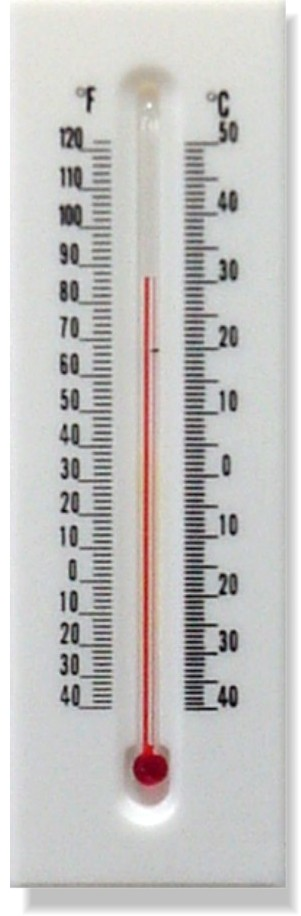Home > Grade 6 > Converting Between Fahrenheit And Celsius

# Converting Between Fahrenheit And Celsius

Directions: When is the value of the temperature in Fahrenheit the same as the value of the temperature in Celsius?

### Hint

How can we demonstrate this using numbers (a table of values), pictures (looking at a thermometer that uses both units), algebra, and words?

### Answer

The answer is -40º.  In addition to using a table of values or algebra, it can be demonstrated by looking at images of real thermometers like this one:Source: Robert Kaplinsky

## Largest Possible GCF #2

Directions: Using the digits 0-9 at most once, fill in the boxes to make the …

### 2 comments

1.The temperature is always the same as itself regardless of units. It might be better to say the “value of the temperature in degrees Celsius” instead of “temperature in degrees Celsius”.

To see what the problem is, try this analogy: When is the length of someone’s foot in centimeters equal to the length of the foot in millimeters? Length is not a number but a quantity with physical meaning, just like temperature.

•That analogy was super helpful. Thanks for the info. I’ve updated the problem accordingly.